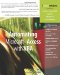# Arrays with Multiple Dimensions

 < Day Day Up >

 Arrays can also use more than one index to locate an element. For example, a two-dimensional array assigns two indexes to each element. To declare a two-dimensional array, you specify the range of each index, separated by commas. For example, this statement defines an array of 10 elements by 10 elements: ` `` Dim arrTaxAmount(1 To 10, 1 To 10) As Double ` When defining or retrieving values from a two-dimensional array, you must supply both index values, as in this procedure: ` `` Public Function GetTaxAmount(intPercent As Integer, _ intAmount As Integer) As Double ' Return a tax amount for the specified ' tax percentage and purchase amount ' Inputs are limited to the range of 1 to 10 Dim i As Integer Dim j As Integer Dim arrTaxAmount(1 To 10, 1 To 10) As Double ' Build the array For i = 1 To 10 For j = 1 To 10 arrTaxAmount(i, j) = (i * j) / 100 Next j Next i ' Now look up the amount GetTaxAmount = arrTaxAmount(intPercent, intAmount) End Function ` Arrays can have up to 60 dimensions, which is far more than you're ever likely to need.
 < Day Day Up >Automating Microsoft Access with VBA
ISBN: 0789732440
EAN: 2147483647
Year: 2003
Pages: 186